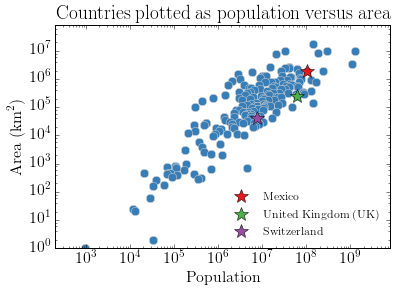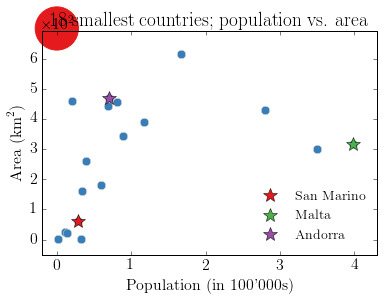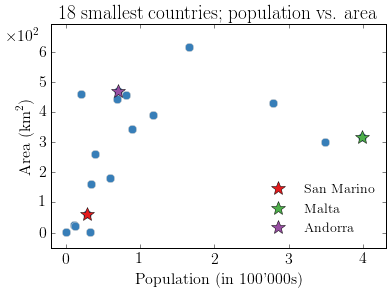# Move scientific notation

#### 18 September 2014

If you plot data with very small or very big amplitudes (plus/minus several orders of magnitude) with Matplotlib it will label the axes in scientific notation. If your small/big data is on the y-scale, the exponent is shown above the y-axes, on the same level as the figure title. There are various reasons why you would like to move this exponent away from where it resides to the side, and in this third entry of my aftershock series I show you my solution.

In my thesis I had many of those figures, as the electromagnetic (EM) responses I dealt with had usually amplitudes to the power of minus ten to zero (minus infinity), so relatively small. However, EM responses might not be in the interest of the average reader, so I show the functionality with some other data. I do therefore some web crawling to get data to plot, something I wanted to try since a long time. To do so I required two more packages, BeautifulSoup and urllib3. BeautifulSoup was already in the anaconda install (bs4), but I still had to install urllib3:

(ipynb) pip install urllib3


## move_sn_y()

The following function, move_sn_y(offs=0, dig=0, side='left', omit_last=False), moves the scientific exponent from the top to the side, either left or right. Possible reasons why you want to move your exponent from the top to the side:

• The exponent overlaps with your figure title.
• Your figure has no title; instead, you include the figure in a document, e.g. $$\LaTeX$$, and create the figure title there. Having the scientific notation on top of the figure creates then unnecessary white space between your title and the actual plot.
• You simply want your figure with scientific notation to appear exactly the same as your figure without it.

For me personally it is a combination of the latter two. Before I get started a word of caution: this function is not terrible stable nor fool-proof. Specifically, it does not thoroughly check the input and all possible cases; expect therefore failures if you do not treat it as it expects you to do.

In :
import urllib3
import numpy as np
from matplotlib import rc
from adashof import circle # To highlight, see Circle.ipynb in this repo
from bs4 import BeautifulSoup
import matplotlib.pyplot as plt

# Increase font size, set CM as default text, and use LaTeX
rc('font', **{'size': 16, 'family': 'serif', 'serif': ['Computer Modern Roman']})
rc('text', usetex=True)

# Define colours (taken from http://colorbrewer2.org)
clr = ['#377eb8', '#e41a1c', '#4daf4a', '#984ea3', '#ff7f00', '#ffff33', '#a65628']


### Load move_sn_y

(You can find it in the notebook adashof.ipynb, in the same repo as this notebook).

In :
%load -s move_sn_y adashof

In :
def move_sn_y(offs=0, dig=0, side='left', omit_last=False):
"""Move scientific notation exponent from top to the side.

Additionally, one can set the number of digits after the comma
for the y-ticks, hence if it should state 1, 1.0, 1.00 and so forth.

Parameters
----------
offs : float, optional; <0>
dig : int, optional; <0>
Number of decimals after the comma.
side : string, optional; {<'left'>, 'right'}
To choose the side of the y-axis notation.
omit_last : bool, optional; <False>
If True, the top y-axis-label is omitted.

Returns
-------
locs : list
List of y-tick locations.

Note
----
This is kind of a non-satisfying hack, which should be handled more
properly. But it works. Functions to look at for a better implementation:
ax.ticklabel_format
ax.yaxis.major.formatter.set_offset_string
"""

# Get the ticks
locs, _ = plt.yticks()

# Put the last entry into a string, ensuring it is in scientific notation
# E.g: 123456789 => '1.235e+08'
llocs = '%.3e' % locs[-1]

# Get the magnitude, hence the number after the 'e'
# E.g: '1.235e+08' => 8
yoff = int(str(llocs).split('e'))

# If omit_last, remove last entry
if omit_last:
slocs = locs[:-1]
else:
slocs = locs

# Set ticks to the requested precision
form = r'$%.'+str(dig)+'f$'
plt.yticks(locs, list(map(lambda x: form % x, slocs/(10**yoff))))

# Define offset depending on the side
if side == 'left':
offs = -.18 - offs # Default left: -0.18
elif side == 'right':
offs = 1 + offs    # Default right: 1.0

# Plot the exponent
plt.text(offs, .98, r'$\times10^{%i}$' % yoff, transform =
plt.gca().transAxes, verticalalignment='top')

# Return the locs
return locs


### Examples showing how to use it

I tried some web crawling for these examples, in order to get some data to plot. The actual plots are not necessarily meaningful, their sole purpose is to show the use of move_sn_y.

In :
# Get some data to plot:
# A list of countries with corresponding population and area
datasite = 'http://www.worldatlas.com/aatlas/populations/ctyareal.htm'
# Worldatlas states the following data sources:
# CIA World Factbook, and other public domain resources (February 2006)

http = urllib3.PoolManager()

# BeautifulSoup is the beast that makes the html better accessible to parse
soup = BeautifulSoup(http.request('GET', datasite).data)

# We are interested in the second table
# (Have a look at the entire source code of the webpage to find out
# which table you need -> print(soup))
table = soup('table')


Now we can parse this table to get the data we are interested in:

In :
# Pre-allocate arrays and list
population = np.zeros(192)
area = np.zeros(192)
country = *192

# Loop through rows and cells to get the information
i = 0
for row in table.findAll("tr"):
cells = row.findAll("td")
# There is a hidden column in which we are not interested, and title columns
# have no text in the first cell, so we can easily exclude them too.
if cells.find(text=True) != None and row['style'] != 'display:none':
# Text in second cell is the country
country[i] = cells.find(text=True)
# Text in third cell is the population; remove the commas to get a numpy-number
population[i] = np.array(cells.find(text=True).replace(',', ''), dtype=float)
# Text in the fourth cell is the area; again, remove the commas
area[i] = np.array(cells.find(text=True).replace(',', ''), dtype=float)
# increase the count
i += 1


First a QC plot to check the data.

In :
# Create figure
fig1 = plt.figure()

# Plot data on a loglog-scale
plt.loglog(population, area, 'o', c=clr, mec='.8', ms=8)

# Highlight some countries
i = 0
for count in ['Mexico', 'United Kingdom (UK)', 'Switzerland']:
ci = country.index(count)
plt.loglog(population[ci], area[ci], '*', c=clr[i+1], mec='k', ms=14, label=count)
i += 1

# Label the plot, set limits, legend
plt.axis([2e2, 8e9, 0, 8e7])
plt.title('Countries plotted as population versus area')
plt.xlabel(r'Population')
plt.ylabel(r'Area ($\rm{km}^2$)')
plt.legend(loc='lower right', frameon=False, fontsize=12, numpoints=1)

plt.show()That looks OK, bigger countries have, generally, more inhabitants. There are a number of prominent outliers, which would be interesting to investigate further, but this is not the purpose of this notebook.

Now I take a subset of the above, namely the 18 smallest countries, and plot again the population versus the area.

In :
# Create figure
fig2a = plt.figure()

# Plot data on a linear scale
plt.plot(population[-18:]/100000, area[-18:], 'o', c=clr, mec='.8', ms=8)

# Highlight some countries
i = 0
for count in ['San Marino', 'Malta', 'Andorra']:
ci = country.index(count)
plt.plot(population[ci]/100000, area[ci], '*', c=clr[i+1], mec='k', ms=14, label=count)
i += 1

# Label the plot, set limits, legend
plt.axis([-.2, 4.3, -50, 690])
plt.title(r'18 smallest countries; population vs. area')
plt.xlabel(r"Population (in 100'000s)")
plt.ylabel(r'Area ($\rm{km}^2$)')
plt.legend(loc='lower right', frameon=False, fontsize=14, numpoints=1)

# Enforce scientific notation
plt.gca().yaxis.major.formatter.set_powerlimits((0,0))

# Highlight the problem
circle((0, 700), .1, {'color':clr, 'clip_on': False})

plt.draw()There we have the issue: the scientific exponent overlaps the title! In this example we could easily solve that by setting a shorter title, of course. But as mentioned in the beginning, you might have other reasons why you do not want the scientific exponent to be on top of the plot.

(Note that in this plot, we could also just label the y-ticks as 0, 100, …, 600, without scientific notation. But I enforced the scientific notation to show the issue.)

In the final figure I do exactly the same as above, but move the scientific exponent with move_sn_y.

In :
# Create figure
fig2b = plt.figure()

# Plot data on a linear scale
plt.plot(population[-18:]/100000, area[-18:], 'o', c=clr, mec='.8', ms=8)

# Highlight some countries
i = 0
for count in ['San Marino', 'Malta', 'Andorra']:
ci = country.index(count)
plt.plot(population[ci]/100000, area[ci], '*', c=clr[i+1], mec='k', ms=14, label=count)
i += 1

# Label the plot, legend
plt.title(r'18 smallest countries; population vs. area')
plt.xlabel(r"Population (in 100'000s)")
plt.ylabel(r'Area ($\rm{km}^2$)')
plt.legend(loc='lower right', frameon=False, fontsize=14, numpoints=1)

# Use move_sn_y to move scientific exponent from top to left
locs = move_sn_y(offs=-.05, side='left')

# Set limits (after move_sn_y)
plt.axis([-.2, 4.3, -50, 690])

plt.draw()There you go, the scientific exponent is on the side of the figure.

The notebook with the above code, MoveSciNot.ipynb, can be found in the usual place, my GitHub blog-notebooks-repo.